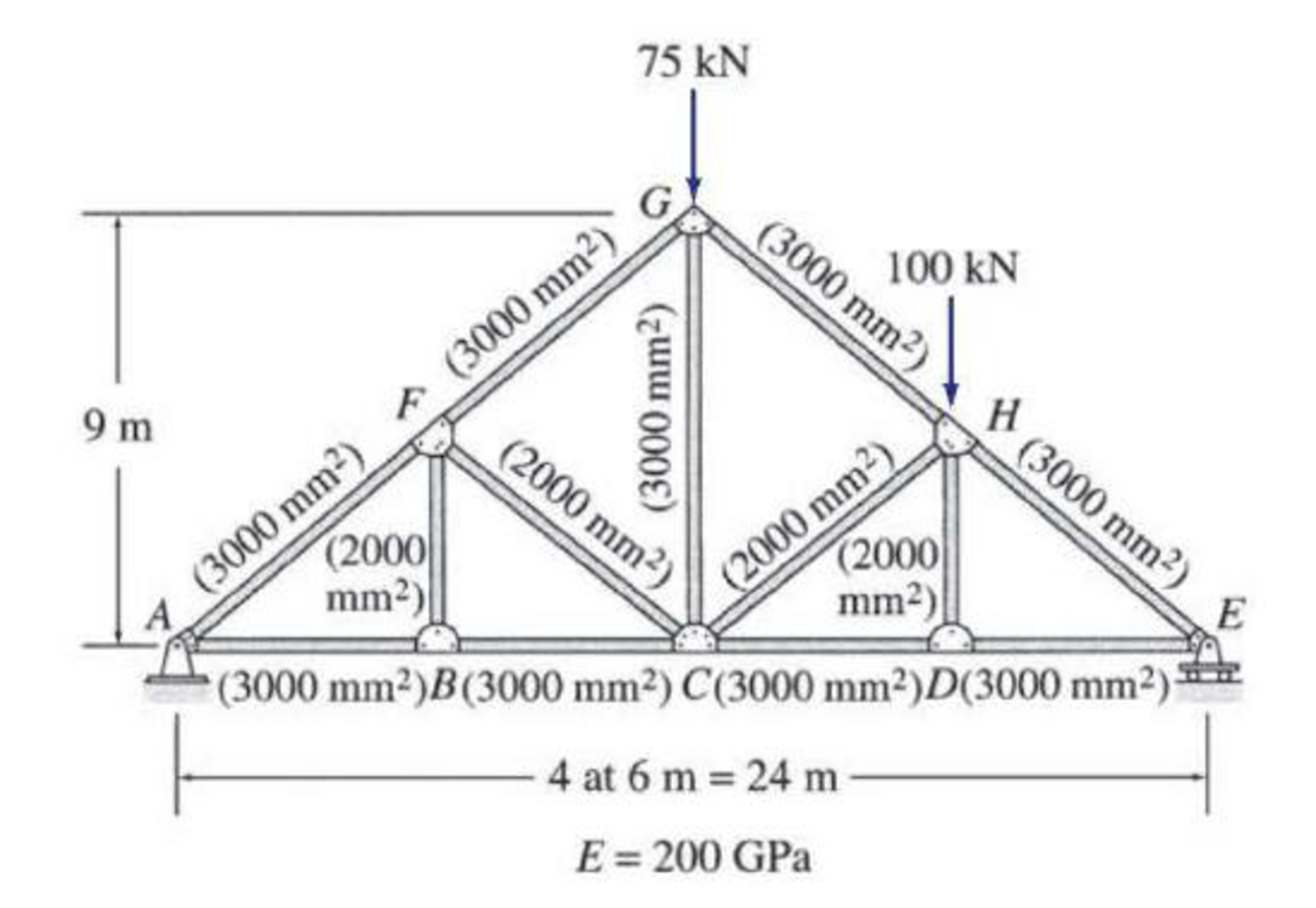# Use the virtual work method to determine the vertical deflection at joint C of the truss shown in Figs. P7.6 and P7.7.

#### Solutions

Chapter
Section
Chapter 7, Problem 7P
Textbook Problem
576 views

## Use the virtual work method to determine the vertical deflection at joint C of the truss shown in Figs. P7.6 and P7.7.To determine

Calculate the vertical deflection at joint C of the truss.

### Explanation of Solution

Given information:

The truss is given in the Figure.

The value of E is 200 GPa.

Procedure to find the deflection of truss by virtual work method is shown below.

• For Real system: If the deflection of truss is determined by the external loads, then apply method of joints or method of sections to find the real axial forces (F) in all the members of the truss.
• For virtual system: Remove all given real loads, apply a unit load at the joint where is deflection is required and also in the direction of desired deflection. Use method of joints or method of sections to find the virtual axial forces (Fv) in all the member of the truss.
• Finally use the desired deflection equation.

Apply the sign conventions for calculating reactions, forces and moments using the three equations of equilibrium as shown below.

• For summation of forces along x-direction is equal to zero (Fx=0), consider the forces acting towards right side as positive (+) and the forces acting towards left side as negative ().
• For summation of forces along y-direction is equal to zero (Fy=0), consider the upward force as positive (+) and the downward force as negative ().
• For summation of moment about a point is equal to zero (Matapoint=0), consider the clockwise moment as negative and the counter clockwise moment as positive.

Method of joints:

The negative value of force in any member indicates compression (C) and the positive value of force in any member indicates tension (T).

Condition for zero force members:

1. 1. If only two non-collinear members are connected to a joint that has no external loads or reactions applied to it, then the force in both the members is zero.
2. 2. If three members, two of which are collinear are connected to a joint that has no external loads or reactions applied to it, then the force in non-collinear member is zero.

Calculation:

Consider the real system.

Find the member axial force (F) for the real system using method of joints:

Let Ax and Ay be the horizontal and vertical reactions at the hinged support A.

Let Ey be the vertical reaction at the roller support E.

Sketch the resultant diagram of the truss as shown in Figure 1.

Find the reactions at the supports using equilibrium equations:

Summation of moments about E is equal to 0.

ME=0Ay(24)75(12)100(6)=0Ay=62.5kN

Summation of forces along y-direction is equal to 0.

+Fy=0Ay+Ey75100=062.5+Ey75100=0Ey=112.5kN

Summation of forces along x-direction is equal to 0.

+Fx=0Ax=0

Find the member forces using method of joints:

The member BF, FC, and DH satisfies the zero force member condition. So the force in the member BF, FC, and DH is zero.

Apply equilibrium equation to the joint A:

Summation of forces along y-direction is equal to 0.

+Fy=062.5+FAFsin36.87°=0FAF=104.17kN

Summation of forces along x-direction is equal to 0.

+Fx=0FAB+FAFcos36.87°=0FAB+(104.17)cos36.87°=0FAB=83.33kN

Apply equilibrium equation to the joint B:

Summation of forces along x-direction is equal to 0.

+Fx=0FAB+FBC=083.33+FBC=0FBC=83.33kN

Apply equilibrium equation to the joint F:

Summation of forces along y-direction is equal to 0.

+Fy=0FFG=FAFFFG=104.17kN

Apply equilibrium equation to the joint E:

Summation of forces along y-direction is equal to 0.

+Fy=0112.5+FEHsin36.87°=0FEH=187.5kN

Summation of forces along x-direction is equal to 0.

+Fx=0FDE+FEHcos36.87°=0FDE+(187.5)cos36.87°=0FDE=150kN

Apply equilibrium equation to the joint D:

Summation of forces along x-direction is equal to 0.

+Fx=0FCD+FDE=0FCD+150=0FCD=150kN

Apply equilibrium equation to the joint C:

Summation of forces along x-direction is equal to 0.

+Fx=0FBC+FCD+FCHcos36.87°=083.33+150+FCHcos36.87°=0FCH=83.33kN

Summation of forces along y-direction is equal to 0.

+Fy=0FCG+FCHsin36.87°=0FCG+(83.33)sin36.87°=0FCG=50kN

Apply equilibrium equation to the joint G:

Summation of forces along x-direction is equal to 0.

+Fx=0FFG=FGHFGH=104.17kN

Consider the virtual system:

For vertical deflection apply l kN at joint C in the vertical direction.

Find the member axial force (Fv) due to virtual vertical load using method of joints:

Sketch the resultant diagram of the virtual system (Fv) as shown in Figure 2.

Find the reactions at the supports using equilibrium equations:

Summation of moments about E is equal to 0.

ME=0Ay(24)1(12)=0Ay=0.5kN

Summation of forces along y-direction is equal to 0.

+Fy=0Ay+Ey1=00

### Still sussing out bartleby?

Check out a sample textbook solution.

See a sample solution

#### The Solution to Your Study Problems

Bartleby provides explanations to thousands of textbook problems written by our experts, many with advanced degrees!

Get Started

Find more solutions based on key concepts
Your car loan payment extends for six years at 8% interest compounded monthly. After how many months do you pay...

Engineering Fundamentals: An Introduction to Engineering (MindTap Course List)

Calculate all forces acting on member CDB.

International Edition---engineering Mechanics: Statics, 4th Edition

What should be done in the case of a personal injury emergency?

Precision Machining Technology (MindTap Course List)

What are ODBC, DAO, and RDO? How are they related?

Database Systems: Design, Implementation, & Management

Explain the uses of and relationship between a document management system (DMS) and a content management system...

Enhanced Discovering Computers 2017 (Shelly Cashman Series) (MindTap Course List)

What is a Tier I ERP software vendor?

Fundamentals of Information Systems

What are XML schema definition (XSD) documents, and what do they do?

Database Systems: Design, Implementation, & Management

What is a milestone, and why is it significant to project planning?

Principles of Information Security (MindTap Course List)

If your motherboard supports ECC DDR3 memory, can you substitute non-ECC DDR3 memory?

A+ Guide to Hardware (Standalone Book) (MindTap Course List)

Describe the differences between pipe and tubing.

Welding: Principles and Applications (MindTap Course List)